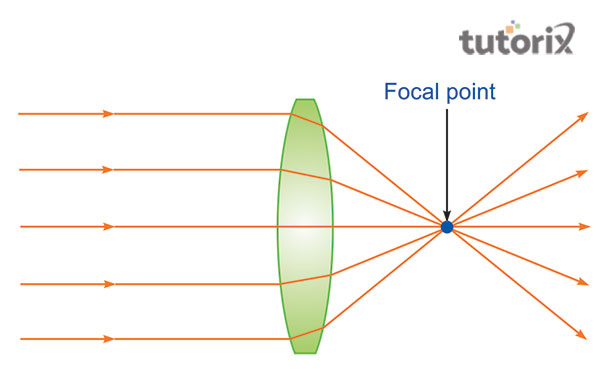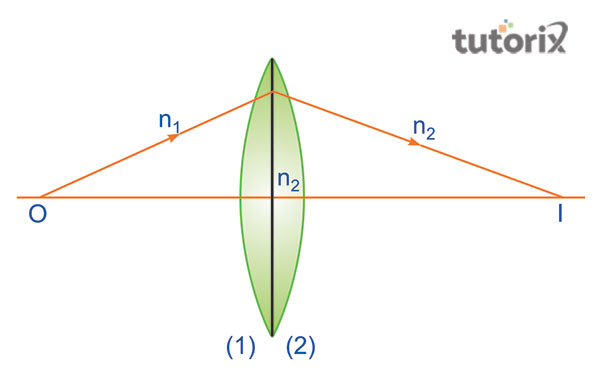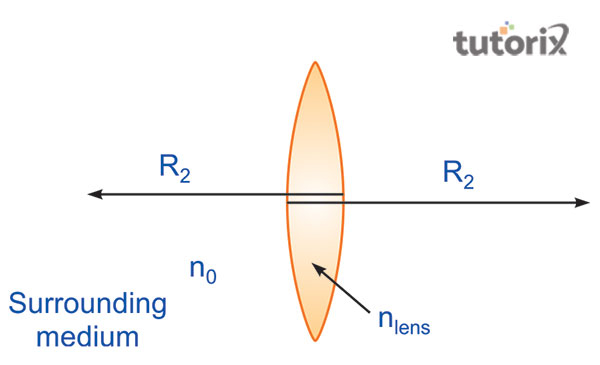# The Lens Makers Formula

## Introduction

The lens maker’s formula is utilized by the lens manufacturers and is used to make lenses of a certain extent of power from a glass of distinct refractive index. It helps the lens makers to derive the necessary data about the curvature that is required to manufacture a lens of a particular focal length in association with the material of a certain refractive index. This formula uses multiple sign conventions which are crucial to set up various geometric relations. This formula is used in the cases of concave and convex lenses alike.

## What is the Lens maker’s Formula?Figure 1: Lens

The lens makers’ formula is generally helpful in forming a relationship between the focal length of a lens and the distinct refractive index of the lens and the radii of curvature of the surfaces. The focal length is dependent on certain factors including the refractive index of the material that is utilized to make the lens and the radius of curvature (phys 2022). This formula is used by manufacturers of lenses to derive an equation that helps make a functional lens that has a distinct relation between focal length, refractive index and radii of curvature of two distinct spheres of certain lenses.

## Conventions of lens makers' formula

There are certain distinct measures associated with the sign conventions that are utilized for making of the lenses. The distance from the optical centre to the right side of the lens is considered to be positive (geeksforgeeks 2022). The length from the optic side of the lens to the left side is negative and all the distances are measured from the optic centre.

The focal length is said to be converging if it is positive and it is diverging if the length is negative.

## Lens maker formula: derivationFigure 2: lens maker’s formula

The derivation of the lens maker formula is obtained in such a manner that, for one surface

n2/v1 -n1/u = n2-n1/R1 …. (1)

For the other surface

n1/v - n2/v1 = n1-n2/r2….. (2)

n1/v - n1/u = (n2- n1) [1/R1 - 1/R2]

= 1/v - 1/u= (n2/n1- 1) [1/R1 - 1/R2]

When, u = ∞ and v =f

1/f = (n2/n1-1) [1/R1 - 1/R2]

Then,

1/v -1/u = 1/f

There it can be established that

1/f = (µ -1) [1/R1 - 1/R2]

In this case, µ is the refractive index of certain material.

## Significance of thickness of the lensFigure 3: lens maker’s formula

The significance of the thickness of lens can be inferred in such a manner that in a case where the ray of light travels through a distinct medium that is air in respect to the medium of the lens. The ray of light undergoes a certain extent of refraction. However, in case the light traverses the lens medium to the medium of air, the light experiences refraction once again (phy-astr 2022).

According to Tsukada et al. (2022, this aspect of double refraction can be avoided with proper usage of the lenses in the optics, that is, the lenses are required to be of thin material that helps in the occurrence of the refraction of light only once. This method is undertaken to ensure the calculations of ray optics are simpler, however, in this process; the first step is generally the identification of the factors that determine the thickness and thinness of the lens.

## Lens maker’s formula: limitations

There are certain limitations associated with this lens maker’s formula that is required to be addressed effectively to obtain the desired result. As opined by Meschede (2017), the lens of the material is needed to be thin it is so to ensure the separation between the two surfaces that refracts light is also required to be small. Accordign to Lien et al. (2022), the medium on either side of the lens is needed to be of the same material. In case a violation is noticed regarding the limitation of this formula then the refraction obtained by the curved surface formula is utilized by both the surfaces.

## Conclusion

The lens maker’s formula is generally used by the manufacturer of lenses to obtain desired results in the production of a lens for each individual. In such a case certain regulations are to be maintained especially the medium of the lens and its associated thickness to avoid a single unit of refraction instead of dual refraction of light. Certain sign conventions are used in the process to ensure correctness in the calculation of the lens maker’s formula, specifically, if the focal length of the lens is derived to be positive then the lens is converging and in case the focal length is negative the lens is concluded to be diverging in nature.

## FAQs

Q1. What is the usage of the lens maker’s formula?

The lens maker’s formula is utilized by the manufacturers of lenses to make lenses of distinct focal lengths. The lenses that have different focal lengths are taken into consideration for the different optical instruments.

Q2. What is a contact lens?

Contact lenses are thin lenses that are specially designed and are placed directly above the viewing place of the eyes. These lenses are won to correct various vision problems and are used for cosmetic and therapeutic purposes as well.

Q3. What are the limitations associated with the lens maker’s formula?

The lenses that are used are required to be thin enough so that the gap between the two distinct surfaces of refractions is small. The medium that is used on both sides of the lens is needed to be same.

Q4. When is the thickness of the lenses ignored and considered?

The thickness of the lens is ignored in case there is no double refraction. However, the thickness is to be rectified in case of the prevalence of double refraction.

Updated on: 18-Aug-2023

333 Views